Next: Theoretical models Up: Odd-even staggering of binding Previous: Introduction

# Odd-even staggering filters

The simplest way to quantify the OES of binding energies is to use the following three-point indicator:(1)

where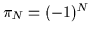is the number parity and B(N) is the (negative) binding energy of a system with N particles. In expression (1), the number of protons Z is fixed, and Ndenotes the number of neutrons; i.e., this indicator gives the neutron OES. An analogous proton OES indicator is obtained by fixing the neutron number N and replacing N by Z in (1).

By applying filter (1) to experimental nuclear binding energies,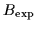, one obtains the experimental neutron and proton OES,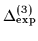. Similarly, by applying this filter to calculated binding energies, one obtains information about theoretical results for the OES. In the following, the same subscripts are used to denote the binding energies and values of indicators (1) obtained for a given model. For example, for the single-particle model described below, the resulting values are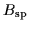and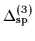. Note that by using filter (1) we only aim at facilitating the comparison of calculations with data; however, in essence we always compare and analyze experimental and calculated binding energies.

As a simple exercise, let us first calculate the OES for a system of particles moving independently in a fixed deformed potential well. In such an extreme single-particle model, the binding energy is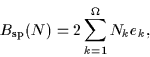(2)

where Nk (=0, 0.5, or 1) and ek stand, respectively, for the occupation number and single-particle energy of the k-th, two-fold degenerate level, and the particle number N is given by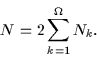(3)

In the above expressions, the single-particle energies ek (k=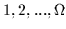) appear in ascending order (e1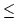e2,...,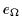), and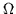denotes the number of twofold degenerate single-particle levels.

For the ground state of an even-N system the N/2 lowest levels are filled. In the neighboring odd-Nsystem, the odd particle occupies the lowest available level. This implies: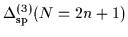= 0 (4)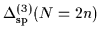=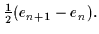(5)

Hence, in the absence of the two-body interaction, filter (1) vanishes at odd particle numbers, and it gives half of the single-particle level spacings at even particle numbers. Therefore, following Ref. , we assume that any other effect leading to the OES beyond the pure single-particle (mean-field) contribution is characterized by values of (1) at odd particle numbers. We also assume that such an effect varies smoothly with the particle number, and, therefore, by subtracting filter (1) at odd and even particle numbers, one may obtain information about the single-particle level spacing at the Fermi level. This hypothesis will be verified in Sec. 3 using several theoretical models.

Consequently, at odd particle numbers N=2n+1, we shall use the following filter,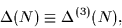(6)

while at even particle numbers N=2n we define,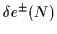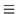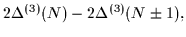(7)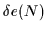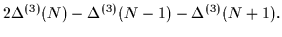(8)

Filters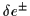correspond to subtracting values of filter (1) at particle numbers higher or lower by one, while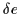employs the symmetric average of both. The differences between these three filters reflect, therefore, small variations of the OES effects with particle numbers. Up to these small variations, we associate the values of these filters with the spacings of the single-particle levels,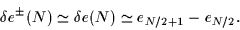(9)

It is clear that filtersuse masses of four nuclides near the given particle number N; i.e., they constitute asymmetric expressions in which either heavier or lighter nuclides dominate. On the other hand,uses masses of five nuclides symmetrically on both sides of N. The advantages of using either of these filters depend, therefore, predominantly on the availability of experimental data in the isotopic or isotonic chains. For instance, in Ref.  dealing with light- and medium-mass nuclei, the filter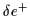was discussed.

It is instructive to relate the asymmetric energy-spacing filtersof Eq. (8) to the particle separation energies S(N)=B(N-1)-B(N), i.e.,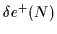= S(N) - S(N+2), (10)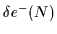= S(N-1) - S(N+1). (11)

One sees that the filterdepends on separation energies of particles from even systems, while the filter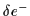depends on those from odd systems. This does not involve any asymmetry in treating even and odd systems, because, obviously, every particle-separation energy depends on one mass of an even system and on one mass of an odd system.

By using the three-mass filter (6), we hope to avoid mixing the contributions to the OES which originate from single-particle structure with those having other roots, e.g., pairing correlations. Moreover, because it involves three masses only, this filter allows obtaining experimental information on the longest isotopic or isotonic chains. Figures 1 and 2 display experimental values of the neutron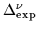and proton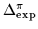OES (6). (It is to be noted that these results slightly differ from those presented in Ref. . Firstly, experimental masses were taken from an updated mass evaluation . Secondly, only the masses having an experimental uncertainty less than 100keV have been considered.)

It is seen that, especially for the light- and medium-mass nuclei, there exists a substantial spread of results around the average trend. This suggests that neutron and proton OES effects are not only functions of neutron and proton numbers, respectively, but that a significant cross-talk between both types of nucleons exists. Numerous studies of this isotopic dependence of the OES exist [24,25,26], usually based on higher-order filters such as the four-point mass formula [21,22,24]: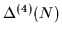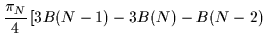+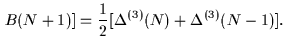(12)

Unfortunately, as demonstrated in Ref. , the higher-order filters mix the pairing and single-particle contributions to the OES; hence, they are not very useful for the purpose of extracting the pairing component. Since the detailed analysis of the isotopic dependence of the three-mass filter results is not yet available, below we discuss the average trends; i.e., for each N the values of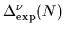are averaged over all isotones for which the data exist, and, similarly, for each Z the values of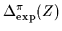are averaged over all isotopes.

Figure 3 (and solid lines in Figs. 1 and 2) present such average values,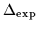, for the neutron and proton OES. Neutron and proton values of the OES follow a similar pattern. Namely, they systematically decrease with A, and they are reduced around shell and subshell closures, as expected. It is also seen that neutron pairing gaps are systematically larger than the proton ones. The shift is mainly due to a mass dependence of the pairing strength and results from the fact that at a given value N, data are more available for lighter nuclei than at an identical value of Z. Indeed, for N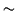Z, data exist mostly at A(N)<A(Z). A weak contribution from the Coulomb energy is also expected to contribute to this shift.Next: Theoretical models Up: Odd-even staggering of binding Previous: Introduction
Jacek Dobaczewski
2000-03-09